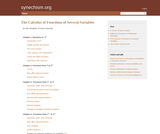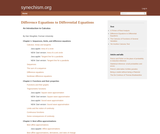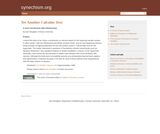# Search Results (6)

View
Selected filters:
• Furman UniversityConditions of Use:
Remix and Share
Rating

This books covers the following topics: Geometry of Rn; functions from R to Rn; functions from Rn to R; functions from Rm to Rn.

Subject:
Calculus
Material Type:
Homework/Assignment
Textbook
Provider:
Furman University
Author:
Dan Sloughter
02/16/2011Conditions of Use:
Remix and Share
Rating

This book covers the following topics: Sequences, limits, and difference equations; functions and their properties; best affine approximations; integration; polynomial approximations and Taylor series; transcendental functions; the complex plane; differential equations.

Subject:
Mathematics
Material Type:
Textbook
Provider:
Furman University
Author:
Sloughter, Dan
02/16/2011Conditions of Use:
Remix and Share
Rating

In his analysis of inference into three types, deduction, induction, and abduction, C. S. Peirce maintains that probability plays an essential role in the first two, but not in the third. For a deductive argument, probability tells us the frequency with which the conclusion will hold given the premises; for an inductive argument, probability tells us the frequency with which the argument will hold true. However, probability has no role to play in abduction because there is, in Peirce's view, no common ground between the conclusion and the premises on which to base a calculation of frequencies. Nevertheless, there is something in the argument that makes the conclusion plausible to our mind. I will argue that this something is a probability which is, in many cases, quantifiable. This probability is the significance level of the statistical logic developed by R. A. Fisher in the 1920's.

Subject:
Statistics and Probability
Material Type:
Provider:
Furman University
Author:
Dan Sloughter
02/16/2011Conditions of Use:
Remix and Share
Rating

A discussion of an alternative version of one of Zeno's paradoxes, and its connection to the nature of the continuum and the existence of infinitesimals. (Unpublished paper)

Subject:
Mathematics
Material Type:
Provider:
Furman University
Author:
Dan Sloughter
02/16/2011Conditions of Use:
Remix and Share
Rating

A discussion of how small probabilities license statistical inferences, and how frequentists C. S. Peirce, R. A. Fisher, J. Neyman, E. S. Pearson, and D. Mayo differ in their interpretations of the p-value of a statistical test. (Unpublished paper)

Subject:
Statistics and Probability
Material Type:
Provider:
Furman University
Author:
Sloughter, Dan
02/16/2011Conditions of Use:
Remix and Share
Rating

I intend this book to be, firstly, a introduction to calculus based on the hyperreal number system. In other words, I will use infinitesimal and infinite numbers freely. Just as most beginning calculus books provide no logical justification for the real number system, I will provide none for the hyperreals. The reader interested in questions of foundations should consult books such as Abraham Robinson's Non-standard Analysis or Robert Goldblatt's Lectures on the Hyperreals. Secondly, I have aimed the text primarily at readers who already have some familiarity with calculus. Although the book does not explicitly assume any prerequisites beyond basic algebra and trigonometry, in practice the pace is too fast for most of those without some acquaintance with the basic notions of calculus.

Subject:
Calculus
Material Type:
Textbook
Provider:
Furman University
Author:
Dan Sloughter